# RS Aggarwal Solutions Class 10 Ex 18D

## RS Aggarwal Class 10 Ex 18D Chapter 18

The RS Aggarwal Class 10 Solutions Chapter 18 Areas Of Circle Sector And Segment Ex 18D (18.4) have been formulated in accordance with the latest syllabus of the CBSE, which makes these solutions highly effective from exam point of view. These solutions not only help students improve their understanding of difficult concepts, rather they give students the ability to solve difficult questions with ease. Using these solutions, you can greatly improve your question-solving abilities and perform exceptionally well in the exam.

If you want to accelerate your exam preparation and score good marks, then these RS Aggarwal Class 10 Solutions can help you great deal. It also helps you grasp important topics quickly and develop a strong foundation for the exam. It will help you to tackle difficult and lengthy questions and score good marks in the exam.

## Download PDF of RS Aggarwal Class 10 Solutions Chapter 18 Areas Of Circle Sector And Segment Ex 18D (18.4)

FAQs

Q.1: The side of a square is 10 cm. Find

(i) The area of the inscribed circle, and

(ii) The area of the circumscribed circle.  [Take $\pi = 3.14$ ]

Sol:

(i) If a circle is inscribed in a square, then the side of the square is equal to the diameter of the circle.

Side of the square = 10 cm

Side = Diameter = 10

$Therefore,$ Radius = 5 cm

Area of the inscribed circle = $\pi r^{2}$ = $3.14 \times 5 \times 5$ = 78.5 $cm^{2}$

(ii) If a circle is circumscribed in a square, then the diagonal of the square is equal to the diameter of the circle.

Diagonal of the square = $\sqrt{2} \times$ Side of the square

$= \sqrt{2} \times 10$

$= 10 \sqrt{2}$ cm

Diagonal = Diameter = $= 10 \sqrt{2}$ cm

$Therefore, r = 5 \sqrt{2}$ cm

Now, Area of the circumscribed circle = $\pi r^{2}$

$= 3.14 \times (5\sqrt{2})^{2}$ = $3.14 \times 50$ = 157 $cm^{2}$

Q.2: If a square is inscribed in a circle, find the ratio of the areas of the circle and the square.

Sol:

If a square is inscribed in a circle, then the diagonals of the square are diameters of the circle.

Let the diagonal of the square be d cm.

Thus, we have:

Radius, r = $\frac{d}{2}$ cm

Area of the circle = $\pi r^{2}$ = $\pi \frac{d^{2}}{4} \; cm^{2}$

We know:

d = $\sqrt{2} \times Side$

$\Rightarrow Side = \frac{d}{\sqrt{2}} \; cm$

Area of the square = $( Side) ^{2}$

$= \left ( \frac{d}{\sqrt{2}} \right ) ^{2}$

$= \left ( \frac{d^{2}}{2} \right ) \; cm^{2}$

Ratio of the area of the circle to that of the square :

$= \frac{\pi \frac{d^{2}}{4}}{\frac{d^{2}}{2}}$ = $\frac{\pi}{2}$

Thus, the ratio of the area of the circle to that of the square is $\pi : 2$

Q.3: The area of a circle inscribed in an equilateral triangle is 154 $cm^{2}$. Find the perimeter of the triangle.  [Take $\sqrt{3} = 1.73$ ]

Sol:

Let the radius of the inscribed circle be r cm.

Given:

Area of the circle = 154 $cm^{2}$

We know:

Area of the circle = $\pi r^{2}$

$\Rightarrow 154 = \frac{22}{7} r^{2}$

$\Rightarrow r^{2} = \frac{154 \times 7}{22}$

$\Rightarrow r^{2} = 49$

$\Rightarrow r = 7$

In a triangle, the centre of the inscribed circle is the point of intersection of the median and the altitudes of the triangle. The centroid divides the median of a triangle in the ratio 2:1.

Here, AO: OD = 2 : 1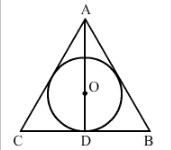Now, Let the altitude be h cm.

We have:

$\angle ADB = 90^{\circ}$

OD = $\frac{1}{3} AD$

$OD = \frac{h}{3}$

$\Rightarrow h = 3r$

$\Rightarrow h = 21$

Let each side of the triangle be a cm.

In the right- angled triangle ADB, we have:

$AB^{2} = AD^{2} + DB^{2}$

$a^{2} = h^{2} + \left ( \frac{a}{2} \right )^{2}$

$3a^{2} = 4h^{2}$

$a^{2} = \frac{ 4h^{2}}{3}$

$a= \frac{2h}{\sqrt{3}}$

$a= \frac{42}{\sqrt{3}}$

$Therefore,$ Perimeter of the triangle = 3a

$= 3 \times \frac{42}{\sqrt{3}}$

$= \sqrt{3} \times 42$ = 72.66 cm

Q.4: The radius of the wheels of a vehicle is 42 cm. How many revolutions will it complete in a 19.8 km long journey?

Sol:

Radius of the wheels = 42 cm

Circumference of the wheels = $2 \pi r$

$2 \times \frac{22}{7} \times 42$ = 264 cm = 2.64 m

Distance covered by the wheel in 1 revolution = 2.64 m

Total distance = 19.8 km or 19800 m

$Therefore,$ Number of revolutions taken by the wheel $= \frac{19800}{2.64} = 7500$

Q.5: The wheels of the locomotive of a train are 2.1 m in radius. They make 75 revolutions in one minute. Find the speed of the train in km per hour.

Sol:

Radius of the wheels = 2.1 m

Circumference of the wheels = $2 \pi r$

$2 \times \frac{22}{7} \times 2.1$ = 13.2 m

Distance covered by the wheels in 1 revolution = 13.2 m

Distance covered by the wheels in 75 revolutions $= \left ( 13.2 \times 75 \right ) = \left ( 990 \times \frac{1}{1000} \right ) km$

Distance covered by the wheel in 1 minute = Distance covered by the wheels in 75 revolutions $= \frac{990}{1000}km$

$Therefore,$ Distance covered by the wheels in 1 hour $= \frac{990}{1000} \times 60$ = 59.4 km/h

Q.6: The wheels of a car makes 2500 revolutions in covering a distance of 4.95 km. Find the diameter of a wheel.

Sol:

Distance = 4.95 km $= 4.95 \times 1000 \times 100$ cm

$Therefore,$ Distance covered by the wheels in 1 revolution = $\frac{Total \; distance \; covered }{Number \; of \; revolutions}$

$\frac{4.95 \times 1000 \times 100}{2500} = 198$ cm

Now,

Circumference of the wheel = 198 cm

$\Rightarrow 2 \pi r =198$

$\Rightarrow 2 \times \frac{22}{7} \times r =198$

$\Rightarrow r = \frac{198 \times 7}{44}$ = 31.5 cm

$Therefore,$ Diameter of the wheel = 2r = 2 (31.5) = 63 cm

Q.7: A boy is cycling in such a way that the wheels of his bicycle are making 14 revolutions per minute. If the diameter of a wheel is 60 cm, calculate the speed (in km/h) at which the boy is cycling.

Sol:

Diameter of the wheel = 60 cm

$Therefore,$ Radius of the wheels = 30 cm

Circumference of the wheels = $2 \pi r$

= $2 \times \frac{22}{7} \times 30$ = $\frac{1320}{7}$ cm

Distance covered by the wheel in 1 revolution = $\frac{1320}{7}$ cm

$Therefore,$ Distance covered by the wheel in 140 revolution = $\left ( \frac{1320}{7} \times 140 \times \frac{1}{100} \right )$ m

= $\left ( \frac{1320 \times 140}{7 \times 100} \times \frac{1}{1000} \right ) \; km = \frac{264}{1000} \; km$

Now,

Distance covered by the wheel in 1 minute = Distance covered by the wheel in 140 revolution $= \frac{264}{1000} \; km$

$Therefore,$ Distance covered by the wheel in 1 hour = $= \frac{264}{1000} \times 60 = 15.84 \; km/h$

Hence, the speed at which the boy is cycling is 15.84 km/h

Q.8: The diameter of the wheels of a bus is 140 cm. How many revolutions per minute do the wheels make when the bus is moving at a speed of 72.6 km/h.

Sol:

Diameter of the wheels = 140 cm

Circumference = $2 \pi r$

$= 2 \times \frac{22}{7} \times 70$ = 440 cm

Speed of the wheels = 72.6 km /h

Distance covered by the wheels in 1 minute = $= \frac{76.2 \times 1000 \times 100}{60} = 121000$ cm

Number of revolutions made by the wheels in 1 minute = $= \frac{Total \; distance \; covered}{Circumference }$

$= \frac{121000}{440}$ = 275

Hence, the wheel makes 275 revolutions per minute.

Q.9: The diameters of the front and rear wheels of a tractor are 80 cm and 2 m respectively. Find the number of revolutions that a rear wheel makes to cover the distance which the front wheel covers in 800 revolutions.

Sol:

Radius of front wheel = 40 cm = $\frac{2}{5}$ m

Circumference of the front wheel = $= \left ( 2 \pi \times \frac{2}{5} \right ) m = \frac{4 \pi}{5}$

Distance covered by the front wheel in 800 revolutions = $= \left ( \frac{4 \pi}{5}\times 800 \right ) m = \left (640 \pi \right ) m$

Radius of the rear wheel = 1 m

Circumference of the rear wheel $= \left (2 \pi \times 1\right ) = 2 \pi\; m$

$Therefore,$ Required number of revolution = $\frac{Distance \; covered \; by \; the \; front \; wheel \; in \; 800 \; revolutions}{Circumference \; of \; the \; rear \; wheel}$

$=\frac{640 \pi }{2 \pi}$ =320

Q.10: Four equal circles are described about the four corners of a square so that each touches woe of the others, as shown in the figure. Find the area of the shaded region, if each side of the square measures 14 cm.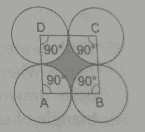Sol:

Side of the square = 14 cm

Radius of the circle = $= \frac{14}{2} = 7$ cm

Area of the quadrant of one circle $= \frac{1}{4} \times \frac{22}{7} \times 7 \times 7$ =38.5 $cm^{2}$

Area of the quadrant of four circles = $38.5 \times 4 = 154 cm^{2}$

Now,

Area of the square = $(Side)^{2}$ = $(14)^{2}$ = 196 $cm^{2}$

Area of the shaded region = Area of the square -Area of the quadrants of the four circles = 196 – 154 = 42 $cm^{2}$

Q.11: Four equal circles, each of radius 5 cm , touch each other, as shown in the figure. Find the area included between them.  [Take $\pi = 3.14$ ]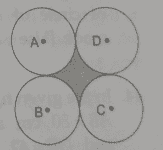Sol: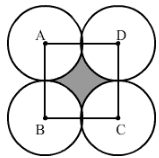AB= BC= CD= AC = 10 cm

All sides are equal , so it is a square.

Area of a square = $Side^{2}$

Area of a square = $10^{2}= 100 \; cm^{2}$

Area of the quadrant of one circle = $= \frac{1}{4} \pi r^{2}$

= $= \frac{1}{4} \times \frac{22}{7} \times 5 \times 5$ = 19.64 $cm^{2}$

Area of the quadrant of four circles = $19.64 \times 4 = 78.57 \; cm^{2}$

Area of the shaded portion = Area of the square – Area of the quadrant of four circles

= 100 – 78.57 = 21.43 $cm^{2}$

Q.12: Four circles, each of radius a units, touch each other. Show that the area between them is $\left (\frac{6}{7} a^{2} \right )$ sq. units .

Sol: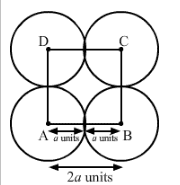When four circles touches each other, their centres from the vertices of a square. The sides of the square are 2a units.

Area of the square = $(2a)^{2}= 4a^{2}$ sq. units

Area occupied by the four sectors = $4 \times \frac{90}{360} \times \pi \times a^{2}$

= $\pi aa^{2}$ sq. units

Area between the circles = Area of the square – Area of the four sector

$= \left ( 4 – \frac{22}{7} \right ) a^{2}$

$= \left ( \frac{6}{7} \right ) a^{2}$ sq. units

Q.13: Three equal circles, each of radius 6 cm, touch one another as shown in the figure. Find the area enclosed between them.  [$\pi = 3.14 \; and \; \sqrt{3} =1.732$]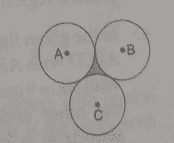Sol: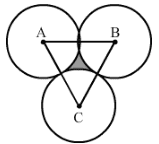Join ABC, all sides are equal, so it is the equilateral triangle.

Now, Area of the equilateral triangle $= \frac{\sqrt{3}}{4} \times Side^{2}$

$= \frac{1.73}{4} \times 12 \times 12$

= 62.28 $cm^{2}$

Area of the arc of the circle = $= \frac{60}{360} \pi r^{2}$

$= \frac{1}{6} \times \frac{22}{7} \times 6 \times 6$ = 18.86 $cm^{2}$

Area of the three sectors $= 3 \times 18.86 = 56.57 \; cm^{2}$

Area of the shaded portion = Area of the triangle – Area of the three quadrant

= 62.28 – 56.57 = 5.71 $cm^{2}$

Q.14: If three circles of radius a each, are drawn such that each touches the other two, prove that the area included between them is equal to $\frac{4}{5} a^{2}$.   [$\pi = 3.14 \; and \; \sqrt{3} =1.732$ ]

Sol: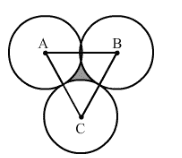When three circles touch each other, their centres from an equilateral triangle, with each side being 2a.

Area of the triangle = $\frac{\sqrt{3}}{4} \times 2a \times 2a = \sqrt{3}a^{2}$

Total area of the three sectors of circles $=3 \times \frac{60}{360} \times \frac{22}{7} \times a^{2} = \frac{1}{2} \times \frac{22}{7} a^{2} = \frac{11}{7} a^{2}$

Area of the region between the circles = Area of the triangle – Area of the three sectors

$= \left ( \sqrt{3} – \frac{11}{7} \right ) a^{2}$

$= \left ( 1.73 – 1.57 \right ) a^{2}$

$= 0.16 a^{2}$

$= \frac{4}{25} a^{2}$

Q.15: In the given figure, ABCD is a trapezium of area 24.5 $cm^{2}$. If $AD \parallel BC$, $\angle DAB = 90^{\circ}$, AD = 10 cm and ABE is quadrant of a circle then find the area of the shaded region.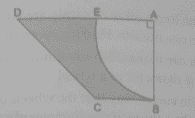Sol:

Area of trapezium = $\frac{1}{2} (AD + BC) \times AB$

$\Rightarrow 24.5 = \frac{1}{2} (10+4) \times AB$

$\Rightarrow AB = 3.5$ cm

Area of the shaded region = Area of trapezium ABCD – Area of quadrant ABE

= 24.5 – $\frac{1}{4} \pi (AB)^{2}$

$= 24.5 – \frac{1}{4} \times \frac{22}{7} \times (3.5)^{2}$

= 24.5 – 9.625 = 14.875 $cm^{2}$

Hence, the area of shaded region is 14.875 $cm^{2}$.

Q.16: ABCD is a field in the shape of trapezium $AD \parallel BC, \angle ABC = 90^{\circ} \;and \; \angle ADC = 60^{\circ}$. Four sectors are formed with centres A,B,C and D, as shown in the figure. The radius of each sector is 14 m. Find the following

(i) Total area of the four sectors,

(ii) Area of the remaining portion, given that AD = 55 m ,  BC = 45 m and AB = 30 m.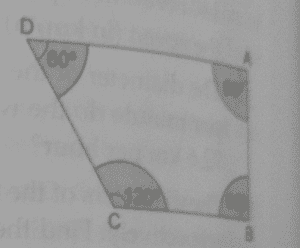Sol:

(i) Area of four sectors = Area of sector having central angle $60^{\circ}$ + Area of sectors having central angle $90^{\circ}$ + Area of sectors having central angle $120^{\circ}$

$\frac{60^{\circ}}{360^{\circ}} .\pi (14)^{2} + \frac{90^{\circ}}{360^{\circ}}. \pi (14)^{2} + \frac{90^{\circ}}{360^{\circ}} .\pi (14)^{2} + \frac{120^{\circ}}{360^{\circ}} .\pi (14)^{2}$

$\left ( \frac{60^{\circ}}{360^{\circ}} + \frac{90^{\circ}}{360^{\circ}} + \frac{90^{\circ}}{360^{\circ}} + \frac{120^{\circ}}{360^{\circ}} \right ) \pi (14)^{2}$

$\left ( \frac{360^{\circ}}{360^{\circ}} \right ) .\pi (14)^{2}$

$= 616 m^{2}$

(ii) Area of the remaining portion = Area of trapezium ABCD – Area of four quadrants

$= \frac{1}{2}(AD+BC) \times AB – 616$

$= \frac{1}{2}(55+45) \times 30 – 616$

= 1500 – 616 = 884 $m^{2}$

Q.17: Find the area of the shaded region in the given figure, where a circulation arc of radius 6 cm has been drawn with the vertex of an equilateral triangle of side 12 cm as the centre and a sector of the circle of radius 6 cm with centre B is made. [ Use $\sqrt {3}=1.73 \; and \; \pi = 3.14$]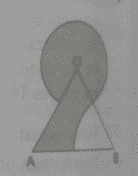Sol:

In equilateral triangle all the angles are of $60^{\circ}$

$Therefore, \angle ABO = \angle AOB = 60^{\circ}$

Area of the shaded region = ( Area of triangle – Area of sector having central angle $60^{\circ}$ + Area of sector having central angle ( $360^{\circ} – 60^{\circ}$ )

= $\frac{\sqrt{3}}{4}(AB)^{2} – \frac{60}{360} . \pi (6)^{2} + \frac{300}{360}. \pi (6)^{2}$

$= \frac{\sqrt{1.73}}{4}(12)^{2} – \frac{1}{6}\times 3.14 (6)^{2} + \frac{5}{6} \times 3.14 (6)^{2}$

= 62.28 – 18.84 + 94.2

= 137.64 $cm^{2}$

Hence, the area of shaded region is 137.64 $cm^{2}$.

Q.18: In the given figure, ABCD is a rectangle with AB = 80 cm and BC = 70 cm,$\angle AED = 90^{\circ}$ and DE = 42 cm. A semicircle is drawn, taking BC  as diameter. Find the area of the shaded region.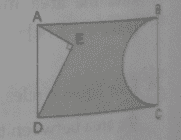Sol:

We know that the opposite sides of a rectangle are equal.

In right triangle AED

$AE^{2}= AD^{2}- DE^{2}$

$AE^{2}= 70^{2}- 12^{2}$

$= 4900 – 1764$ = 3136

$\Rightarrow AE= 56$

Area of the shaded region = Area of rectangle – (Area of triangle + Area of semicircle )

= $AB \times BC – \left [ \frac{1}{2} \times AE \times DE +\frac{1}{2} \pi \left ( \frac{BC}{2} \right )^{2}\right ]$

$= 80 \times 70 – \left [ \frac{1}{2} \times 56 \times 42 +\frac{1}{2} \times \frac{22}{7} \times \left ( \frac{70}{2} \right )^{2} \right ]$

= 5600 – 3101 = 2499 $cm^{2}$

Hence, the area of shaded region is 2499 $cm^{2}$

### Key Features of RS Aggarwal Class 10 Solutions Chapter 18 Areas Of Circle Sector And Segment Ex 18D (18.4)

• It gives you confidence to solve all types of questions asked in the exam.
• It covers the entire syllabus as per the CBSE.
• It provides different methods to solve difficult and tricky questions.
• It is the best resource for the students when it comes to revision.

#### Practise This Question

The magnetic field line passing through the centre of a circular current carrying conductor is